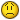# generate automatically sum

L

#### Luchian

I have 5 colomn with 6 row with number 1 or 0

A B C D E
0 1 0 1 1
1 0 0 1 1
1 1 1 0 0
0 0 0 0 0
0 1 1 0 0
1 1 1 0 1

It's possibile to generate in column F all possibile results if sum one cell
in every row. If i make simple formula (add one cell in everry row, one by
one) will look like that:

F
A1+A2+A3+A4+A5+A6
A1+A2+A3+A4+A5+B6
....
A1+A2+A3+B4+B5+B6
....
B1+A2+A3+A4+B5+B6
....
B1+C2+A3+D4+B5+E6
....
E1+E2+E3+E4+E5+E6

It was 15625 (5^) formulas to insert . Can i GENERATE ALL THIS SUMS
automatically?

S

#### Susan

well, manually you could type in cell F1

=sum(a1:e1)

and then use the fill handle to drag it down your 15000 rows. it will

you could also use a macro. right click on your sheet tab, choose
"view code" and then paste this:

Sub Luchian()

Dim myRange As Range
Dim c As Range

Set myRange = ActiveSheet.Range("f1:f15625")

For Each c In myRange
c.Formula = "=sum(a" & c.Row & ":e" & c.Row & ")"
Next c

End Sub

hope it helps!susan

S

#### Susan

well, never mind. i think i did not comprehend what you want to do.
maybe it will give you an idea, though.susan

L

#### Luchian

Well, it's not what i want, but you give me a clue with macro. Thank you

"Susan" a scris:

P

#### Pete_UK

Here's a way of doing it by a single formula in F1 and then copied
down.

To check that it works, though, put these simpler formulae in the
cells stated:

G1: =CHAR(65+MOD(INT((ROW()-1)/5/5/5/5/5),5))&"1"
H1: =CHAR(65+MOD(INT((ROW()-1)/5/5/5/5),5))&"2"
I1: =CHAR(65+MOD(INT((ROW()-1)/5/5/5),5))&"3"
J1: =CHAR(65+MOD(INT((ROW()-1)/5/5),5))&"4"
K1: =CHAR(65+MOD(INT((ROW()-1)/5),5))&"5"
L1: =CHAR(65+MOD(ROW()-1,5))&"6"

If you copy those down you will get this in the first few rows:

A1 A2 A3 A4 A5 A6
A1 A2 A3 A4 A5 B6
A1 A2 A3 A4 A5 C6
A1 A2 A3 A4 A5 D6
A1 A2 A3 A4 A5 E6
A1 A2 A3 A4 B5 A6
A1 A2 A3 A4 B5 B6
A1 A2 A3 A4 B5 C6
A1 A2 A3 A4 B5 D6
A1 A2 A3 A4 B5 E6
A1 A2 A3 A4 C5 A6
A1 A2 A3 A4 C5 B6
A1 A2 A3 A4 C5 C6

and if you copy all the way to row 15625 you will find all
combinations of the cell references.

So, all we need to do is to combine those into one formula and get
Excel to convert the strings into cell references. Put this in F1:

=INDIRECT(CHAR(65+MOD(INT((ROW()-1)/5/5/5/5/5),5))&"1")
+INDIRECT(CHAR(65+MOD(INT((ROW()-1)/5/5/5/5),5))&"2")
+INDIRECT(CHAR(65+MOD(INT((ROW()-1)/5/5/5),5))&"3")
+INDIRECT(CHAR(65+MOD(INT((ROW()-1)/5/5),5))&"4")
+INDIRECT(CHAR(65+MOD(INT((ROW()-1)/5),5))&"5")
+INDIRECT(CHAR(65+MOD((ROW()-1),5))&"6")

and then you can copy this down to F15625. You can delete columns G to
L.

Hope this helps.

Pete

L

#### Luchian

That it! Thank you!!!

"Pete_UK" a scris:
Here's a way of doing it by a single formula in F1 and then copied
down.

To check that it works, though, put these simpler formulae in the
cells stated:

G1: =CHAR(65+MOD(INT((ROW()-1)/5/5/5/5/5),5))&"1"
H1: =CHAR(65+MOD(INT((ROW()-1)/5/5/5/5),5))&"2"
I1: =CHAR(65+MOD(INT((ROW()-1)/5/5/5),5))&"3"
J1: =CHAR(65+MOD(INT((ROW()-1)/5/5),5))&"4"
K1: =CHAR(65+MOD(INT((ROW()-1)/5),5))&"5"
L1: =CHAR(65+MOD(ROW()-1,5))&"6"

If you copy those down you will get this in the first few rows:

A1 A2 A3 A4 A5 A6
A1 A2 A3 A4 A5 B6
A1 A2 A3 A4 A5 C6
A1 A2 A3 A4 A5 D6
A1 A2 A3 A4 A5 E6
A1 A2 A3 A4 B5 A6
A1 A2 A3 A4 B5 B6
A1 A2 A3 A4 B5 C6
A1 A2 A3 A4 B5 D6
A1 A2 A3 A4 B5 E6
A1 A2 A3 A4 C5 A6
A1 A2 A3 A4 C5 B6
A1 A2 A3 A4 C5 C6

and if you copy all the way to row 15625 you will find all
combinations of the cell references.

So, all we need to do is to combine those into one formula and get
Excel to convert the strings into cell references. Put this in F1:

=INDIRECT(CHAR(65+MOD(INT((ROW()-1)/5/5/5/5/5),5))&"1")
+INDIRECT(CHAR(65+MOD(INT((ROW()-1)/5/5/5/5),5))&"2")
+INDIRECT(CHAR(65+MOD(INT((ROW()-1)/5/5/5),5))&"3")
+INDIRECT(CHAR(65+MOD(INT((ROW()-1)/5/5),5))&"4")
+INDIRECT(CHAR(65+MOD(INT((ROW()-1)/5),5))&"5")
+INDIRECT(CHAR(65+MOD((ROW()-1),5))&"6")

and then you can copy this down to F15625. You can delete columns G to
L.

Hope this helps.

Pete

P

#### Pete_UK

You're welcome - thanks for feeding back.

Incidentally, what did you need this for?

Pete